Pair distribution function

For a fluid of$N$ particles, enclosed in a volume$V$ at a given temperature$T$ (canonical ensemble) interacting via the `central' intermolecular pair potential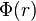$\Phi(r)$, the two particle distribution function is defined as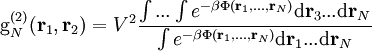${\rm g}_N^{(2)}({\mathbf r}_1,{\mathbf r}_2)= V^2 \frac{\int ... \int e^{-\beta \Phi({\mathbf r}_1,...,{\mathbf r}_N)}{\rm d}{\mathbf r}_3...{\rm d}{\mathbf r}_N}{\int e^{-\beta \Phi({\mathbf r}_1,...,{\mathbf r}_N)}{\rm d}{\mathbf r}_1...{\rm d}{\mathbf r}_N}$

where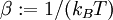$\beta := 1/(k_BT)$, where$k_B$ is the Boltzmann constant.

Exact convolution equation for${\mathrm g}(r)$

See Eq. 5.10 of Ref. 1: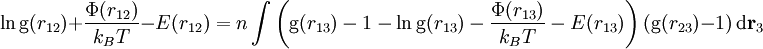$\ln {\mathrm g}(r_{12}) + \frac{\Phi(r_{12})}{k_BT} - E(r_{12}) = n \int \left({\mathrm g}(r_{13}) -1 - \ln {\mathrm g}(r_{13}) - \frac{\Phi(r_{13})}{k_BT} - E(r_{13}) \right)({\mathrm g}(r_{23}) -1) ~{\rm d}{\mathbf r}_3$

where, i.e.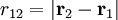$r_{12} = |{\mathbf r}_2 - {\mathbf r}_1|$.